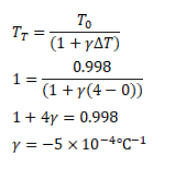# The density of water atQuestion:

The density of water at $0^{\circ} \mathrm{C}$ is $0.998 \mathrm{~g} / \mathrm{cm}^{3}$ and at $4^{\circ} \mathrm{C}$ is $1.00 \mathrm{~g} / \mathrm{cm}^{3}$. Calculate the average coefficient of volume expansion of water in the temperature range 0 to $4^{\circ} \mathrm{C}$.

Solution: